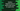# Python program to find out the sum of odd and even numbers in a list# Introduction :

In this python programming tutorial, we will learn how to find the sum of all odd numbers and even numbers in a list. The program will ask the user to enter the size of the list first. Then, it will ask each number to add to the list one by one. Finally, the program will calculate and print out the sum of all odd numbers and even numbers in the list.

## Algorithm :

We will use the below algorithm to solve this problem :

1. Ask the user to enter the list size.
2. Take all inputs of the list from the user one by one.
3. Now, calculate the sum of all odd numbers and even numbers in the list.
4. Finally, print out the sum of odd numbers and even numbers.

### Python program :

``````#1
size = int(input("Enter the size of the list : "))

#2
sum_odd = 0
sum_even = 0

#3
int_list = []

#4
for i in range(size):
#5
n = int(input("Enter element {} : ".format(i+1)))
int_list.append(n)

#6
for i in range(size):
#7
if(int_list[i] % 2 == 0):
sum_even += int_list[i]
else:
sum_odd += int_list[i]

#8
print("Sum of odd numbers : {} ".format(sum_odd))
print("Sum of even numbers : {} ".format(sum_even))``````

### Explanation :

The commented numbers in the above program denote the step numbers below :

1. Ask the user to enter the size of the list. Read it and store it in the size variable.
2. Create two variables sumodd_ and sumeven_ to hold the sum of odd and even numbers in the list.
3. Create one empty list intlist_.
4. Run one for loop in the range of the user provided size.If the size is 4 this loop will run for_ 4 times starting i = 0 to i = 3_.
5. On each iteration of the loop, ask the user to enter the element for the list. Read each element and append it to the list intlist_.
6. Run one more loop similarly in the same range. This loop is for finding out the sum of odd and even numbers in the list.
7. On each iteration, check if the current iterating element of the list is_ even or odd. If even, add it to the variable _sumeven_ and if odd, add it to sumodd_.
8. Finally, print out the sum of all odd and even numbers to the user.

### Sample Output :

``````Enter the size of the list : 3
Enter element 1 : 1
Enter element 2 : 2
Enter element 3 : 3
Sum of odd numbers : 4
Sum of even numbers : 2

Enter the size of the list : 5
Enter element 1 : 12
Enter element 2 : 23
Enter element 3 : 34
Enter element 4 : 22
Enter element 5 : 1
Sum of odd numbers : 24
Sum of even numbers : 68``````

### Conclusion :

As you have in the example, we can easily calculate the sum of odd and even numbers in a list in python. Try to run the example above and drop one comment below if you have any queries.